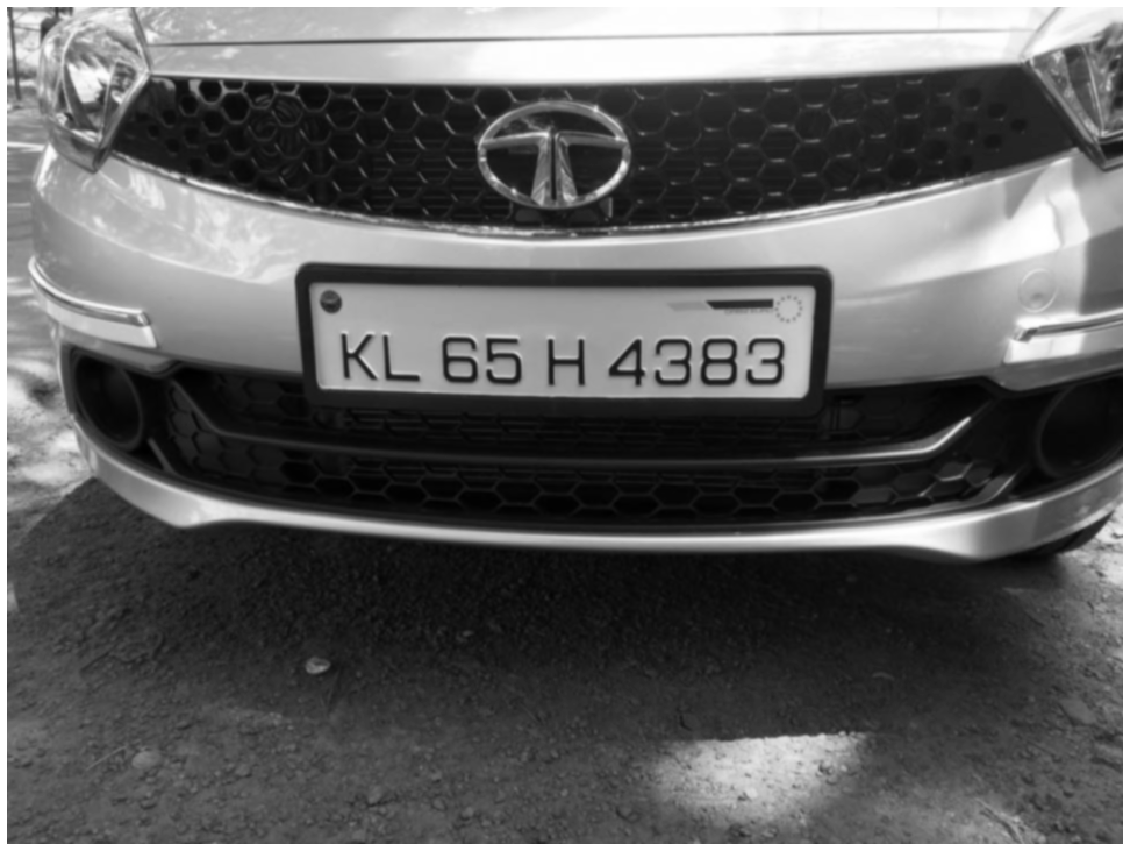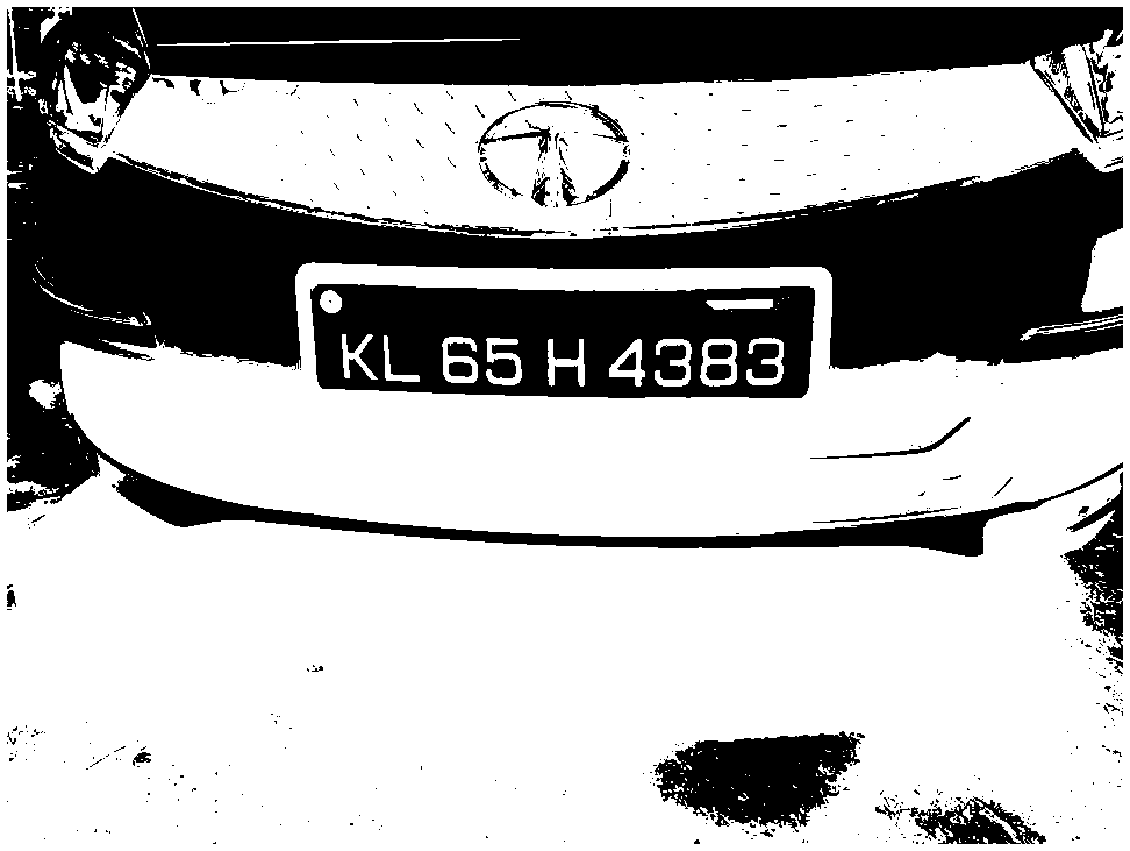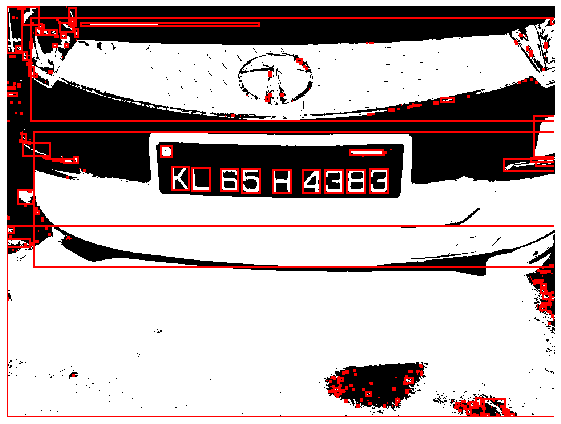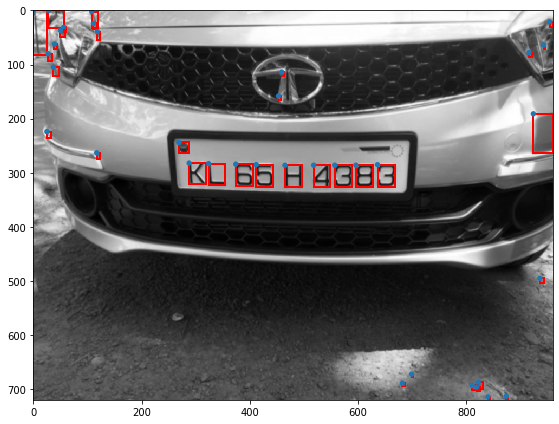In :
import matplotlib.pyplot as plt
import numpy as np
from skimage.color import rgb2gray
from skimage.filters import threshold_otsu
from skimage.filters import gaussian

# read the image and convert it to grayscale
gray_img = rgb2gray(car)

#blur the image to remove any noise
blurred_gray_img = gaussian(gray_img)
plt.figure(figsize=(20,20))
plt.axis("off")
plt.imshow(blurred_gray_img, cmap="gray")

Out:
<matplotlib.image.AxesImage at 0x7f152a10e978>## Binarize the image to seperate the foreground from background.¶

In :
import matplotlib.patches as mpatches
from skimage.measure import label, regionprops
from skimage.util import invert
from scipy.spatial.distance import euclidean

thresh = threshold_otsu(gray_img)
print(thresh)
thresh = 0.46
binary = invert(gray_img > thresh)

plt.figure(figsize=(20,20))
plt.axis("off")
plt.imshow(binary, cmap="gray")

0.45194776731004904

Out:
<matplotlib.image.AxesImage at 0x7f1529c47f28>## Connected component analysis of the image. connectivity set to 2 for 8 connected regions.¶

In :
label_image = label(binary, connectivity=2)

fig, ax = plt.subplots(figsize=(10, 6))
ax.axis("off")
ax.imshow(binary, cmap="gray")

for region in regionprops(label_image):
minr, minc, maxr, maxc = region.bbox
rect = mpatches.Rectangle((minc, minr), maxc - minc, maxr - minr,
fill=False, edgecolor='red', linewidth=2)

plt.tight_layout()
plt.show()## Identify regions which probably contains text.¶

In :
fig, ax = plt.subplots(figsize=(10, 6))
ax.imshow(blurred_gray_img, cmap="gray")

text_like_regions = []
for region in regionprops(label_image):
minr, minc, maxr, maxc = region.bbox
w = maxc - minc
h = maxr - minr

asr = w/h

region_area = w*h

wid,hei = blurred_gray_img.shape
img_area = wid*hei

# The aspect ratio is less than 1 to eliminate highly elongated regions
# The size of the region should be greater than 15 pixels but smaller than 1/5th of the image
# dimension to be considered for further processing
if region_area > 15 and region_area < (0.2 * img_area) and asr < 1 and h > w:
#print(w, h, i, region.area, region.bbox)
text_like_regions.append(region)

all_points = []
for region in text_like_regions:
minr, minc, maxr, maxc = region.bbox
rect = mpatches.Rectangle((minc, minr), maxc - minc, maxr - minr,
fill=False, edgecolor='red', linewidth=2)
all_points.append([minc, minr, maxc, maxr])

plt.tight_layout()
plt.show()In :
# find the angle between the three points A,B,C
# Angle between line BA and BC
def angle_between_three_points(pointA, pointB, pointC):
BA = pointA - pointB
BC = pointC - pointB

try:
cosine_angle = np.dot(BA, BC) / (np.linalg.norm(BA) * np.linalg.norm(BC))
angle = np.arccos(cosine_angle)
except:
print("exc")
raise Exception('invalid cosine')

return np.degrees(angle)

all_points = np.array(all_points)

all_points = all_points[all_points[:,1].argsort()]
height, width = blurred_gray_img.shape
groups = []
for p in all_points:
cluster = [p]
lines_found = False
for q in all_points:
pmin = np.array([p,p])
qmin = np.array([q,q])
if p < q and euclidean(pmin,qmin) < 0.1 * width:
for cpoints in cluster:
if cpoints == q and cpoints == q:
break;
cluster.append(q)

for r in all_points:
rmin = np.array([r,r])
last_cluster_point = np.array([cluster[-1],cluster[-1]])
if q < r and euclidean(last_cluster_point,rmin) < 0.1 * width:
angle = angle_between_three_points(pmin,qmin,rmin)
if angle > 170 and angle < 190:
lines_found = True
cluster.append(r)

if lines_found:
groups.append(np.array(cluster))

# plot the longest found line on the image
longest = -1
longest_index = -1
for index, cluster in enumerate(groups):
if len(cluster) > longest:
longest_index = index
longest = len(cluster)

print("coordinates of licence plate\n")
print(groups[longest_index])

fig, ax = plt.subplots(figsize=(10, 6))
ax.imshow(blurred_gray_img, cmap="gray")
for region in groups[longest_index]:
minc = region
minr = region
maxc = region
maxr = region
rect = mpatches.Rectangle((minc, minr), maxc - minc, maxr - minr,
fill=False, edgecolor='red', linewidth=2)
plt.tight_layout()
plt.show()

coordinates of licence plate

[[288 282 319 321]
[324 283 355 323]
[374 284 405 324]
[412 285 443 326]
[465 286 497 327]
[518 286 549 327]
[596 286 628 327]
[557 286 589 327]
[465 286 497 327]
[518 286 549 327]]